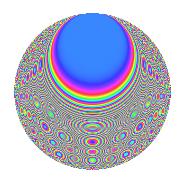# Properties

 Label 3024.2.csLevel 3024 Weight 2 Character orbit cs Rep. character $$\chi_{3024}(271,\cdot)$$ Character field $$\Q(\zeta_{6})$$ Dimension 128 Sturm bound 1152

# Related objects

## Defining parameters

 Level: $$N$$ = $$3024 = 2^{4} \cdot 3^{3} \cdot 7$$ Weight: $$k$$ = $$2$$ Character orbit: $$[\chi]$$ = 3024.cs (of order $$6$$ and degree $$2$$) Character conductor: $$\operatorname{cond}(\chi)$$ = $$28$$ Character field: $$\Q(\zeta_{6})$$ Sturm bound: $$1152$$

## Dimensions

The following table gives the dimensions of various subspaces of $$M_{2}(3024, [\chi])$$.

Total New Old
Modular forms 1224 128 1096
Cusp forms 1080 128 952
Eisenstein series 144 0 144

## Trace form

 $$128q + O(q^{10})$$ $$128q + 52q^{25} - 4q^{37} + 8q^{49} - 12q^{73} - 48q^{85} + O(q^{100})$$

## Decomposition of $$S_{2}^{\mathrm{new}}(3024, [\chi])$$ into newform subspaces

The newforms in this space have not yet been added to the LMFDB.

## Decomposition of $$S_{2}^{\mathrm{old}}(3024, [\chi])$$ into lower level spaces

$$S_{2}^{\mathrm{old}}(3024, [\chi]) \cong$$ $$S_{2}^{\mathrm{new}}(28, [\chi])$$$$^{\oplus 12}$$$$\oplus$$$$S_{2}^{\mathrm{new}}(84, [\chi])$$$$^{\oplus 9}$$$$\oplus$$$$S_{2}^{\mathrm{new}}(112, [\chi])$$$$^{\oplus 4}$$$$\oplus$$$$S_{2}^{\mathrm{new}}(252, [\chi])$$$$^{\oplus 6}$$$$\oplus$$$$S_{2}^{\mathrm{new}}(336, [\chi])$$$$^{\oplus 3}$$$$\oplus$$$$S_{2}^{\mathrm{new}}(756, [\chi])$$$$^{\oplus 3}$$$$\oplus$$$$S_{2}^{\mathrm{new}}(1008, [\chi])$$$$^{\oplus 2}$$

## Hecke Characteristic Polynomials

There are no characteristic polynomials of Hecke operators in the database